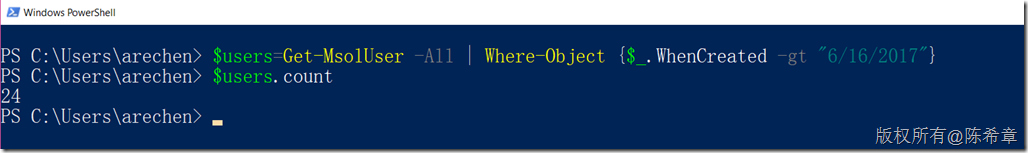# Office 365 批量生成用户

最近更新时间：2018-05-28

## CSV 文件说明。

```DisplayName UserPrincipalName Department Password UsageLocation

```

```随机生成数字：=INT(RAND()*10)

=CONCATENATE(IF(INT(RAND()*2)=0,CHAR(INT(RAND()*9+48)),IF(INT(RAND()*2)=0,CHAR(INT(RAND()*25+65)),CHAR(INT(RAND()*25+97)))),IF(INT(RAND()*2)=0,CHAR(INT(RAND()*9+48)),IF(INT(RAND()*2)=0,CHAR(INT(RAND()*25+65)),CHAR(INT(RAND()*25+97)))),IF(INT(RAND()*2)=0,CHAR(INT(RAND()*9+48)),IF(INT(RAND()*2)=0,CHAR(INT(RAND()*25+65)),CHAR(INT(RAND()*25+97)))),IF(INT(RAND()*2)=0,CHAR(INT(RAND()*9+48)),IF(INT(RAND()*2)=0,CHAR(INT(RAND()*25+65)),CHAR(INT(RAND()*25+97)))),IF(INT(RAND()*2)=0,CHAR(INT(RAND()*9+48)),IF(INT(RAND()*2)=0,CHAR(INT(RAND()*25+65)),CHAR(INT(RAND()*25+97)))),IF(INT(RAND()*2)=0,CHAR(INT(RAND()*9+48)),IF(INT(RAND()*2)=0,CHAR(INT(RAND()*25+65)),CHAR(INT(RAND()*25+97)))))
```

```DisplayName	UserPrincipalName	Department	Password	UsageLocation
teyixa	        [email protected]	EDU	        af2T3qdO4	CN
```

## 使用说明(pwershell)

```#导入office模块
Import-module MSOnline
#登录office，必须有365开号权限
\$Credential = Get-Credential
#链接
connect-msolservice -credential \$Credential
#查询授权
Get-MsolAccountSku
```

```Import-Csv -Path “D:\user.csv” | ForEach {New-MsolUser -DisplayName \$_.DisplayName -UserPrincipalName \$_.UserPrincipalName -Department \$_.Department -Password \$_.Password -UsageLocation \$_.UsageLocation -LicenseAssignment ‘XXXXXXXXXXX’ -PasswordNeverExpires \$true -ForceChangePassword \$False}
```

## 批量删除用户

### 操作

[-City ] [-Country ] [-Department ] [-DomainName ] [-EnabledFilter ][-SearchString ]

```Where-Object {\$_.WhenCreated –gt “6/16/2017”}
``````\$users.count
\$users | Remove-MsolUser –Force
```